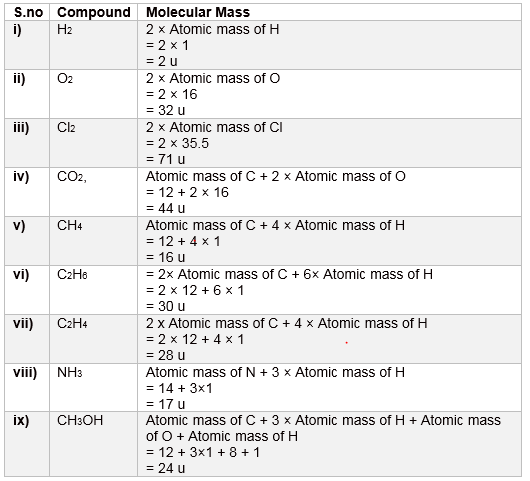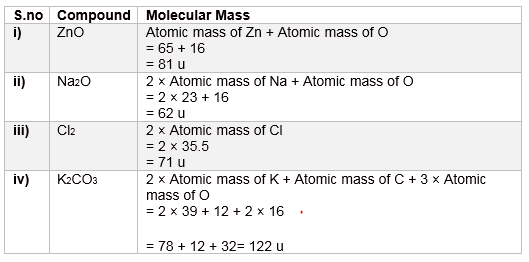# NCERT Solutions for Class9 Science: Chapter 3 Atoms and Molecules

In this page we have NCERT Solutions for Class9 Science: Chapter 3 Atoms and Molecules . Hope you like them and do not forget to like , social shar and comment at the end of the page.

## NCERT intext Solutions

Pages 32-33
Question 1.
In a reaction, 5.3 g of sodium carbonate reacted with 6 g of ethanoic acid. The products were 2.2 g of carbon dioxide, 0.9 g water and 8.2 g of sodium ethanoate. Show that these observations are in agreement with the law of conservation of mass.
Sodium carbonate + ethanoic acid → sodium ethanoate + carbon dioxide + water
In the given reaction, sodium carbonate reacts with ethanoic acid to produce sodium ethanoate, carbon dioxide, and water.
Sodium Carbonate + Ethanoic acid → sodium ethanoate + carbon dioxide + water
Mass of sodium carbonate = 5.3 g (Given)
Mass of ethanoic acid = 6 g (Given)
Mass of sodium ethanoate = 8.2 g (Given)
Mass of carbon dioxide = 2.2 g (Given)
Mass of water = 0.9 g (Given)
Now, total mass before the reaction = (5.3 + 6) g
= 11.3 g
And, total mass after the reaction = (8.2 + 2.2 + 0.9) g
= 11.3 g
∴ Total mass before the reaction = Total mass after the reaction
Hence, the given observations are in agreement with the law of conservation of mass.

Question 2.
Hydrogen and oxygen combine in the ratio of 1:8 by mass to form water. What mass of oxygen gas would be required to react completely with 3 g of hydrogen gas?
It is given that the ratio of hydrogen and oxygen by mass to form water is 1:8.
Then, the mass of oxygen gas required to react completely with 1 g of hydrogen gas is 8 g.
Therefore, the mass of oxygen gas required to react completely with 3 g of hydrogen gas is 8 × 3 g = 24 g.

Question 3.
Which postulate of Dalton's atomic theory is the result of the law of conservation of mass?
The postulate of Dalton: “Atoms are indivisible particles, which cannot be created or destroyed in a chemical reaction" is the result of the law of conservation of mass.

Question 4.
Which postulate of Dalton's atomic theory can explain the law of definite proportions?
The postulate of Dalton, “The relative number and kinds of atoms are constant in a given compound”, can explain the law of definite proportions.

Pages 36
Question 1.
Define atomic mass unit.
Mass unit equal to exactly one-twelfth the mass of one atom of carbon-12 is called one atomic mass unit. It is written as 'u'.

Question 2.
Why is it not possible to see an atom with naked eyes?
The size of an atom is so small that it is not possible to see it with naked eyes. Also, the atom of an element does not exist independently.

Pages 39
Question 1.
Write down the formulae of
(i) sodium oxide
(ii) aluminium chloride
(iii) sodium sulphide
(iv) magnesium hydroxide
 (i) sodium oxide $Na_2O$ (ii) aluminium chloride $AlCl_3$ (iii) sodium sulphide $Na_2S$ (iv) magnesium hydroxide $Mg(OH)_2$

Question 2.
Write down the names of compounds represented by the following formulae:
(i) $Al_2(SO_4)_3$
(ii) $CaCl_2$
(iii) $K_2SO_4$
(iv) $KNO_3$
(v) $CaCO_3$
 (i) $Al_2(SO_4)_3$ Aluminium sulphate (ii) $CaCl_2$ Calcium chloride (iii) $K_2SO_4$ Potassium sulphate (iv) $KNO_3$ Potassium nitrate (v) $CaCO_3$ Calcium carbonate

Question 3.
What is meant by the term chemical formula?
The chemical formula of a compound is a symbolic representation of its composition.
For example
Chemical Formula for water is $H_2O$

Question 4
How many atoms are present in a
(i) $H_2S$ molecule and
(ii) $PO_4^{3-}$ ion?
(i) In an $H_2S$ molecule, three atoms are present; two of hydrogen and one of sulphur.
(ii) In a $PO_4^{3-}$ ion, five atoms are present; one of phosphorus and four of oxygen.
Pages 40
Question 1.
Calculate the molecular masses of $H_2$, $O_2$, $Cl_2$, $CO_2$, $CH_4$, $C_2H_6$, $C_2H_4$, $NH_3$, $CH_3OH$.
Useful information for solving these questions
Atomic mass of H=1
Atomic mass of N=14
Atomic mass of O=16
Atomic mass of Cl=35.5Question 2.
Calculate the formula unit masses of ZnO, Na2O, K2CO3, given atomic masses of Zn = 65 u, Na = 23 u, K = 39 u, C = 12 u, and O = 16 u.Pages 42
Question 1.
If one mole of carbon atoms weighs 12 g, what is the mass (in gram) of 1 atom of carbon?
Given as per the question One mole of carbon atoms weighs 12 g
Now 1 mole=6.022 × 1023 number of carbon atoms
So, mass of 6.022 × 1023 number of carbon atoms = 12 g
Therefore, mass of 1 atom of carbon = 12 / (6.022 × 1023)
= 1.9926 x 10-23 g

Question 2.
Which has more number of atoms, 100 grams of sodium or 100 grams of iron (given, atomic mass of Na = 23 u, Fe = 56 u)?
Atomic mass of Na = 23 u (Given)
Then, gram atomic mass of Na = 23 g
Now, 23 g of Na contains = 6.022 × 1023 g number of atoms
Thus, 100 g of Na contains = 6.022 × 1023 / 23×100 number of atoms
= 2.6182 × 1024 number of atoms
Again, atomic mass of Fe = 56 u (Given)
Then, gram atomic mass of Fe = 56 g
Now, 56 g of Fe contains = 6.022 × 1023 g number of atoms
Thus, 100 g of Fe contains = 6.022 × 1023 / 56 × 100 number of atoms
= 1.0753 × 1024 number of atoms
Therefore, 100 grams of sodium contain more number of atoms than 100 grams of iron.

## NCERT exercises Solutions

Question 1.
A 0.24 g sample of compound of oxygen and boron was found by analysis to contain 0.096 g of boron and 0.144 g of oxygen. Calculate the percentage composition of the compound by weight.
Given as per the question
Total mass of Compound = 0.24 g
Mass of boron = 0.096 g
Mass of oxygen = 0.144 g
So, percentage of boron by weight in the compound = $\frac {0.096}{0.24} \times 100$
= 40%
And, percentage of oxygen by weight in the compound = $\frac {0.144 }{ 0.24 } \times 100$ = 60%

Question 2.
When 3.0 g of carbon is burnt in 8.00 g oxygen, 11.00 g of carbon dioxide is produced. What mass of carbon dioxide will be formed when 3.00 g of carbon is burnt in 50.00 g of oxygen? Which law of chemical combinations will govern your answer?
3.0 g of carbon combines with 8.0 g of oxygen to give 11.0 of carbon dioxide.
If 3 g of carbon is burnt in 50 g of oxygen, then 3 g of carbon will react with 8 g of oxygen. The remaining 42 g of oxygen will be left un-reactive.
In this case also, only 11 g of carbon dioxide will be formed.
The above answer is governed by the law of constant proportions.

Question 3.
What are polyatomic ions? Give examples?
A polyatomic ion is a group of atoms carrying a charge (positive or negative). For example, Nitrate (NO3-) , hydroxide ion (OH - ).

Question 4.
Write the chemical formulae of the following:
(a) Magnesium chloride
(b) Calcium oxide
(c) Copper nitrate
(d) Aluminium chloride
(e) Calcium carbonate
 S.no Compound Chemical Formula a) Magnesium chloride $MgCl_2$ b) Calcium oxide $CaO$ c) Copper nitrate $Cu (NO_3)_2$ d) Aluminium chloride $AlCl_3$ e) Calcium carbonate $CaCO_3$

Question 5.
Give the names of the elements present in the following compounds:
(a) Quick lime
(b) Hydrogen bromide
(c) Baking powder
(d) Potassium sulphate
 a) Quick lime Calcium and oxygen b) Hydrogen bromide Hydrogen and bromine c) Baking powder Sodium, hydrogen, carbon, and oxygen d) Potassium sulphate Potassium, sulphur, and oxygen

Question 6.
Calculate the molar mass of the following substances:
(a) Ethyne, $C_2H_2$
(b) Sulphur molecule, $S_8$
(c) Phosphorus molecule, $P_4$ (atomic mass of phosphorus = 31)
(d) Hydrochloric acid, $HCl$
(e) Nitric acid, $HNO_3$
Given
atomic mass of phosphorus = 31
atomic mass of Sulphur = 32
atomic mass of Chlorine = 35.5
 S.no Compound Molecular Mass (a) Ethyne, $C_2H_2$ 2 × 12 + 2 × 1 = 26 g (b) Sulphur molecule, $S_8$ 8 × 32 = 256 g (c) Phosphorus molecule, $P_4$ 4 × 31 = 124 g (d) Hydrochloric acid, $HCl$ 1 + 35.5 = 36.5 g (e) Nitric acid, $HNO_3$ 1 + 14 + 3 × 16 = 63 g

Question 7.
What is the mass of-
(a) 1 mole of nitrogen atoms?
(b) 4 moles of aluminium atoms (Atomic mass of aluminium = 27)?
(c) 10 moles of sodium sulphite ($Na_2SO_3$)?
(a) As Atomic mass of Nitrogen = 14
So, the mass of 1 mole of nitrogen atoms is 14 g.
(b) As Atomic mass of aluminium = 27
So, the mass of 4 moles of aluminium atoms is (4 × 27) g = 108 g
(c) The mass of 10 moles of sodium sulphite ($Na_2SO_3$) is
$10 \times [2 \times 23 + 32 + 3 \times 16]$ g = 10 × 126 g = 1260 g
Question 8.
Convert into mole.
(a) 12 g of oxygen gas
(b) 20 g of water
(c) 22 g of carbon dioxide
(a) 32 g of oxygen gas = 1 mole
Then, 12 g of oxygen gas = 12 / 32 mole = 0.375 mole
(b)18 g of water = 1 mole
Then, 20 g of water = 20 / 18 mole = 1.111 mole
(c) 44 g of carbon dioxide = 1 mole
Then, 22 g of carbon dioxide = 22 / 44 mole = 0.5 mole
Question 9.
What is the mass of:
(a) 0.2 mole of oxygen atoms?
(b) 0.5 mole of water molecules?
(a) Mass of one mole of oxygen atoms = 16 g
Then, mass of 0.2 mole of oxygen atoms = 0.2 × 16g = 3.2 g
(b) Atomic mass of water= 2×atomic mass of Hydrogen+ atomic mass of oxygen
=2+16=18
So, Mass of one mole of water molecule = 18 g
Then, mass of 0.5 mole of water molecules = 0.5 × 18 g = 9 g

Question 10.
Calculate the number of molecules of sulphur ($S_8$) present in 16 g of solid sulphur.
1 mole of solid sulphur (S8) = 8 × 32 g = 256 g
i.e., 256 g of solid sulphur contains = $6.022 \times 10^{23}$ molecules
Then, 16 g of solid sulphur contains = $\frac {6.022 \times 10^{23}}{256} \times 16$
$= 3.76375 \times 10^{22}$ molecules

Question 11.
Calculate the number of aluminium ions present in 0.051 g of aluminium oxide.
(Hint: The mass of an ion is the same as that of an atom of the same element. Atomic mass of Al = 27 u)
1 mole of aluminium oxide ($Al_2O_3$) = $2 \times 27 + 3 \times 16$
= 102 g
Now, 102 g of $Al_2O_3$= $6.022 \times 10^{23}$ molecules of $Al_2O_3$
Then, 0.051 g of $Al_2O_3$ contains = $\frac {6.022 \times 110^{23}}{102} \times 0.051$ molecules
$= 3.011 \times 10^{20}$ molecules of $Al_2O_3$
The number of aluminium ions ($Al^{3+}$) present in one molecule of aluminium oxide is 2.
Therefore, the number of aluminium ions ($Al^{3+}$ present in $3.011 \times 10^{20}$ molecules (0.051 g) of aluminium oxide ($Al_2O_3$) = $2 \times 3.011 \times 10^{20}$
$= 6.022 \times 10^{20}$